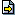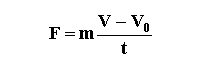Science

Dynamics

 Show figure Without Friction       With Friction Calculate:   Force (F) Weight (P) Mass (m) Acceleration (a) Velocity (V) Time (t) Initial Velocity (V0) = m/s F = N P = N m = kg a = m/s2 V = m/s t = s N = N Fa = N µ =To Memory 01 Memory 02 Memory 03 Memory 04 Memory 05 Memory 06 Replacing Adding Subtracting Multiplying Dividing
 Configurations Maximum of decimal places Gravitational Acceleration (g) = m/s2 Units:   MKS (SI)    MK*S    CGS    English Units Impression Page Title (Optional) Comments to be printed (Optional)